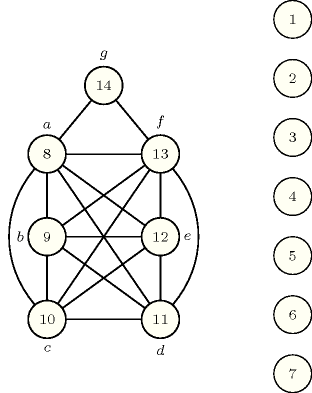## 5.373. stable_compatibility

Origin

P. Flener, [BeldiceanuFlenerLorca06]

Constraint

$\mathrm{𝚜𝚝𝚊𝚋𝚕𝚎}_\mathrm{𝚌𝚘𝚖𝚙𝚊𝚝𝚒𝚋𝚒𝚕𝚒𝚝𝚢}\left(\mathrm{𝙽𝙾𝙳𝙴𝚂}\right)$

Argument
 $\mathrm{𝙽𝙾𝙳𝙴𝚂}$ $\mathrm{𝚌𝚘𝚕𝚕𝚎𝚌𝚝𝚒𝚘𝚗}\left(\begin{array}{c}\mathrm{𝚒𝚗𝚍𝚎𝚡}-\mathrm{𝚒𝚗𝚝},\hfill \\ \mathrm{𝚏𝚊𝚝𝚑𝚎𝚛}-\mathrm{𝚍𝚟𝚊𝚛},\hfill \\ \mathrm{𝚙𝚛𝚎𝚌}-\mathrm{𝚜𝚒𝚗𝚝},\hfill \\ \mathrm{𝚒𝚗𝚌}-\mathrm{𝚜𝚒𝚗𝚝}\hfill \end{array}\right)$
Restrictions
 $\mathrm{𝚛𝚎𝚚𝚞𝚒𝚛𝚎𝚍}$$\left(\mathrm{𝙽𝙾𝙳𝙴𝚂},\left[\mathrm{𝚒𝚗𝚍𝚎𝚡},\mathrm{𝚏𝚊𝚝𝚑𝚎𝚛},\mathrm{𝚙𝚛𝚎𝚌},\mathrm{𝚒𝚗𝚌}\right]\right)$ $\mathrm{𝙽𝙾𝙳𝙴𝚂}.\mathrm{𝚒𝚗𝚍𝚎𝚡}\ge 1$ $\mathrm{𝙽𝙾𝙳𝙴𝚂}.\mathrm{𝚒𝚗𝚍𝚎𝚡}\le |\mathrm{𝙽𝙾𝙳𝙴𝚂}|$ $\mathrm{𝚍𝚒𝚜𝚝𝚒𝚗𝚌𝚝}$$\left(\mathrm{𝙽𝙾𝙳𝙴𝚂},\mathrm{𝚒𝚗𝚍𝚎𝚡}\right)$ $\mathrm{𝙽𝙾𝙳𝙴𝚂}.\mathrm{𝚏𝚊𝚝𝚑𝚎𝚛}\ge 1$ $\mathrm{𝙽𝙾𝙳𝙴𝚂}.\mathrm{𝚏𝚊𝚝𝚑𝚎𝚛}\le |\mathrm{𝙽𝙾𝙳𝙴𝚂}|$ $\mathrm{𝙽𝙾𝙳𝙴𝚂}.\mathrm{𝚙𝚛𝚎𝚌}\ge 1$ $\mathrm{𝙽𝙾𝙳𝙴𝚂}.\mathrm{𝚙𝚛𝚎𝚌}\le |\mathrm{𝙽𝙾𝙳𝙴𝚂}|$ $\mathrm{𝙽𝙾𝙳𝙴𝚂}.\mathrm{𝚒𝚗𝚌}\ge 1$ $\mathrm{𝙽𝙾𝙳𝙴𝚂}.\mathrm{𝚒𝚗𝚌}\le |\mathrm{𝙽𝙾𝙳𝙴𝚂}|$ $\mathrm{𝙽𝙾𝙳𝙴𝚂}.\mathrm{𝚒𝚗𝚌}>\mathrm{𝙽𝙾𝙳𝙴𝚂}.\mathrm{𝚒𝚗𝚍𝚎𝚡}$
Purpose

Enforce the construction of a stably compatible supertree that is compatible with several given trees. The notion of stable compatibility and its context are detailed in the Usage slot.

Example
$\left(\begin{array}{c}〈\begin{array}{cccc}\mathrm{𝚒𝚗𝚍𝚎𝚡}-1\hfill & \mathrm{𝚏𝚊𝚝𝚑𝚎𝚛}-4\hfill & \mathrm{𝚙𝚛𝚎𝚌}-\left\{11,12\right\}\hfill & \mathrm{𝚒𝚗𝚌}-\varnothing ,\hfill \\ \mathrm{𝚒𝚗𝚍𝚎𝚡}-2\hfill & \mathrm{𝚏𝚊𝚝𝚑𝚎𝚛}-3\hfill & \mathrm{𝚙𝚛𝚎𝚌}-\left\{8,9\right\}\hfill & \mathrm{𝚒𝚗𝚌}-\varnothing ,\hfill \\ \mathrm{𝚒𝚗𝚍𝚎𝚡}-3\hfill & \mathrm{𝚏𝚊𝚝𝚑𝚎𝚛}-4\hfill & \mathrm{𝚙𝚛𝚎𝚌}-\left\{2,10\right\}\hfill & \mathrm{𝚒𝚗𝚌}-\varnothing ,\hfill \\ \mathrm{𝚒𝚗𝚍𝚎𝚡}-4\hfill & \mathrm{𝚏𝚊𝚝𝚑𝚎𝚛}-5\hfill & \mathrm{𝚙𝚛𝚎𝚌}-\left\{1,3\right\}\hfill & \mathrm{𝚒𝚗𝚌}-\varnothing ,\hfill \\ \mathrm{𝚒𝚗𝚍𝚎𝚡}-5\hfill & \mathrm{𝚏𝚊𝚝𝚑𝚎𝚛}-7\hfill & \mathrm{𝚙𝚛𝚎𝚌}-\left\{4,13\right\}\hfill & \mathrm{𝚒𝚗𝚌}-\varnothing ,\hfill \\ \mathrm{𝚒𝚗𝚍𝚎𝚡}-6\hfill & \mathrm{𝚏𝚊𝚝𝚑𝚎𝚛}-2\hfill & \mathrm{𝚙𝚛𝚎𝚌}-\left\{8,14\right\}\hfill & \mathrm{𝚒𝚗𝚌}-\varnothing ,\hfill \\ \mathrm{𝚒𝚗𝚍𝚎𝚡}-7\hfill & \mathrm{𝚏𝚊𝚝𝚑𝚎𝚛}-7\hfill & \mathrm{𝚙𝚛𝚎𝚌}-\left\{6,13\right\}\hfill & \mathrm{𝚒𝚗𝚌}-\varnothing ,\hfill \\ \mathrm{𝚒𝚗𝚍𝚎𝚡}-8\hfill & \mathrm{𝚏𝚊𝚝𝚑𝚎𝚛}-6\hfill & \mathrm{𝚙𝚛𝚎𝚌}-\varnothing \hfill & \mathrm{𝚒𝚗𝚌}-\left\{9,10,11,12,13,14\right\},\hfill \\ \mathrm{𝚒𝚗𝚍𝚎𝚡}-9\hfill & \mathrm{𝚏𝚊𝚝𝚑𝚎𝚛}-2\hfill & \mathrm{𝚙𝚛𝚎𝚌}-\varnothing \hfill & \mathrm{𝚒𝚗𝚌}-\left\{10,11,12,13\right\},\hfill \\ \mathrm{𝚒𝚗𝚍𝚎𝚡}-10\hfill & \mathrm{𝚏𝚊𝚝𝚑𝚎𝚛}-3\hfill & \mathrm{𝚙𝚛𝚎𝚌}-\varnothing \hfill & \mathrm{𝚒𝚗𝚌}-\left\{11,12,13\right\},\hfill \\ \mathrm{𝚒𝚗𝚍𝚎𝚡}-11\hfill & \mathrm{𝚏𝚊𝚝𝚑𝚎𝚛}-1\hfill & \mathrm{𝚙𝚛𝚎𝚌}-\varnothing \hfill & \mathrm{𝚒𝚗𝚌}-\left\{12,13\right\},\hfill \\ \mathrm{𝚒𝚗𝚍𝚎𝚡}-12\hfill & \mathrm{𝚏𝚊𝚝𝚑𝚎𝚛}-1\hfill & \mathrm{𝚙𝚛𝚎𝚌}-\varnothing \hfill & \mathrm{𝚒𝚗𝚌}-\left\{13\right\},\hfill \\ \mathrm{𝚒𝚗𝚍𝚎𝚡}-13\hfill & \mathrm{𝚏𝚊𝚝𝚑𝚎𝚛}-5\hfill & \mathrm{𝚙𝚛𝚎𝚌}-\varnothing \hfill & \mathrm{𝚒𝚗𝚌}-\left\{14\right\},\hfill \\ \mathrm{𝚒𝚗𝚍𝚎𝚡}-14\hfill & \mathrm{𝚏𝚊𝚝𝚑𝚎𝚛}-6\hfill & \mathrm{𝚙𝚛𝚎𝚌}-\varnothing \hfill & \mathrm{𝚒𝚗𝚌}-\varnothing \hfill \end{array}〉\hfill \end{array}\right)$

Figure 5.373.1 shows the two trees we want to merge. Note that the leaves $𝚊$ and $𝚏$ occur in both trees.

##### Figure 5.373.1. The two trees to merge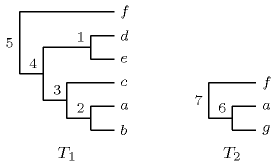The left part of Figure 5.373.2 gives one way to merge the two previous trees. This solution corresponds to the ground instance provided by the example. Note that there exist 7 other ways to merge these two trees. They are respectively depicted by Figures 5.373.2 to 5.373.5.

##### Figure 5.373.2. First solution (corresponding to the ground instance of the example) and second solution on how to merge the two trees ${T}_{1}$ and ${T}_{2}$ of Figure 5.373.1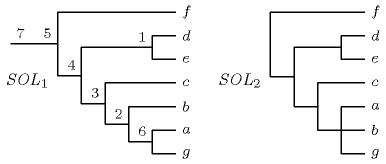##### Figure 5.373.3. Third and fourth solutions on how to merge the two trees ${T}_{1}$ and ${T}_{2}$ of Figure 5.373.1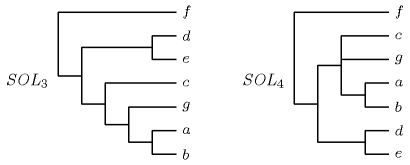##### Figure 5.373.4. Fifth and sixth solutions on how to merge the two trees ${T}_{1}$ and ${T}_{2}$ of Figure 5.373.1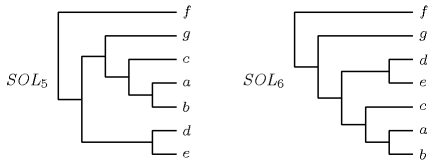##### Figure 5.373.5. Seventh and eight solutions on how to merge the two trees ${T}_{1}$ and ${T}_{2}$ of Figure 5.373.1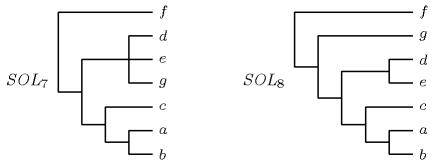Typical
 $|\mathrm{𝙽𝙾𝙳𝙴𝚂}|>2$ $\mathrm{𝚛𝚊𝚗𝚐𝚎}$$\left(\mathrm{𝙽𝙾𝙳𝙴𝚂}.\mathrm{𝚏𝚊𝚝𝚑𝚎𝚛}\right)>1$
Symmetry

Items of $\mathrm{𝙽𝙾𝙳𝙴𝚂}$ are permutable.

Usage

One objective of phylogeny is to construct the genealogy of the species, called the tree of life, whose leaves represent the contemporary species and whose internal nodes represent extinct species that are not necessarily named. An important problem in phylogeny is the construction of a supertree [BinindaEmondsGittlemanSteel02] that is compatible with several given trees. There are several definitions of tree compatibility in the literature:

• A tree $𝒯$ is strongly compatible with a tree ${𝒯}^{\text{'}}$ if ${𝒯}^{\text{'}}$ is topologically equivalent to a subtree $𝒯$ that respects the node labelling. [NgWormald96]

• A tree $𝒯$ is weakly compatible with a tree ${𝒯}^{\text{'}}$ if ${𝒯}^{\text{'}}$ can be obtained from $𝒯$ by a series of arc contractions. [Steel92]

• A tree $𝒯$ is stably compatible with a set $𝒮$ of trees if $𝒯$ is weakly compatible with each tree in $𝒮$ and each internal node of $𝒯$ can be labelled by at least one corresponding internal node of some tree in $𝒮$.

For the supertree problem, strong and weak compatibility coincide if and only if all the given trees are binary [NgWormald96]. The existence of solutions is not lost when restricting weak compatibility to stable compatibility.

##### Figure 5.373.6. Supertree problem instance and two of its solutions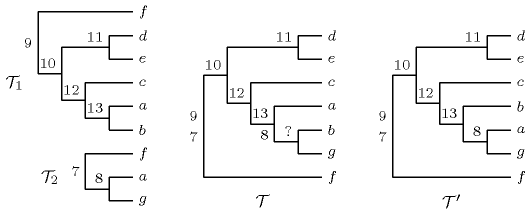##### Figure 5.373.7. Three small phylogenetic trees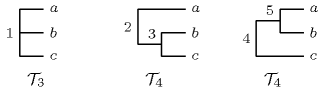For example, the trees ${𝒯}_{1}$ and ${𝒯}_{2}$ of Figure 5.373.6 have $𝒯$ and ${𝒯}^{\text{'}}$ as supertrees under both weak and strong compatibility. As shown, all the internal nodes of ${𝒯}^{\text{'}}$ can be labelled by corresponding internal nodes of the two given trees, but this is not the case for the father of $b$ and $g$ in $𝒯$. Hence $𝒯$ and four other such supertrees are debatable because they speculate about the existence of extinct species that were not in any of the given trees. Consider also the three small trees in Figure 5.373.7: ${𝒯}_{3}$ and ${𝒯}_{4}$ have ${𝒯}_{4}$ as a supertree under weak compatibility, as it suffices to contract the arc $\left(3,2\right)$ to get ${𝒯}_{3}$ from ${𝒯}_{4}$. However, ${𝒯}_{3}$ and ${𝒯}_{4}$ have no supertree under strong compatibility, as the most recent common ancestor of $b$ and $c$, denoted by $\mathrm{𝑚𝑟𝑐𝑎}\left(b,c\right)$, is the same as $\mathrm{𝑚𝑟𝑐𝑎}\left(a,b\right)$ in ${𝒯}_{3}$, namely 1, but not the same in ${𝒯}_{4}$, as $\mathrm{𝑚𝑟𝑐𝑎}\left(b,c\right)=3$ is an evolutionary descendant of $\mathrm{𝑚𝑟𝑐𝑎}\left(a,b\right)=2$. Also, ${𝒯}_{4}$ and ${𝒯}_{5}$ have neither weakly nor strongly compatible supertrees.

Under strong compatibility, a first supertree algorithm was given in [AhoSagivSzymanskiUllman81], with an application for database management systems; it takes $O\left({l}^{2}\right)$ time, where $l$ is the number of leaves in the given trees. Derived algorithms have emerged from phylogeny, for instance OneTree [NgWormald96]. The first constraint program was proposed in [GentProsserSmithWei03], using standard, non-global constraints. Under weak compatibility, a phylogenetic supertree algorithm can be found in [Steel92] for instance. Under stable compatibility, the algorithm from computational linguistics of [BodirskyKutz07] has supertree construction as special case.

Keywords
Arc input(s)

$\mathrm{𝙽𝙾𝙳𝙴𝚂}$

Arc generator
$\mathrm{𝐶𝐿𝐼𝑄𝑈𝐸}$$↦\mathrm{𝚌𝚘𝚕𝚕𝚎𝚌𝚝𝚒𝚘𝚗}\left(\mathrm{𝚗𝚘𝚍𝚎𝚜}\mathtt{1},\mathrm{𝚗𝚘𝚍𝚎𝚜}\mathtt{2}\right)$

Arc arity
Arc constraint(s)
$\mathrm{𝚗𝚘𝚍𝚎𝚜}\mathtt{1}.\mathrm{𝚏𝚊𝚝𝚑𝚎𝚛}=\mathrm{𝚗𝚘𝚍𝚎𝚜}\mathtt{2}.\mathrm{𝚒𝚗𝚍𝚎𝚡}$
Graph property(ies)
 $•$$\mathrm{𝐌𝐀𝐗}_\mathrm{𝐍𝐒𝐂𝐂}$$\le 1$ $•$$\mathrm{𝐍𝐂𝐂}$$=1$ $•$$\mathrm{𝐌𝐀𝐗}_\mathrm{𝐈𝐃}$$\le 2$ $•$$\mathrm{𝐏𝐀𝐓𝐇}_\mathrm{𝐅𝐑𝐎𝐌}_\mathrm{𝐓𝐎}$$\left(\mathrm{𝚒𝚗𝚍𝚎𝚡},\mathrm{𝚒𝚗𝚍𝚎𝚡},\mathrm{𝚙𝚛𝚎𝚌}\right)=1$ $•$$\mathrm{𝐏𝐀𝐓𝐇}_\mathrm{𝐅𝐑𝐎𝐌}_\mathrm{𝐓𝐎}$$\left(\mathrm{𝚒𝚗𝚍𝚎𝚡},\mathrm{𝚒𝚗𝚍𝚎𝚡},\mathrm{𝚒𝚗𝚌}\right)=0$ $•$$\mathrm{𝐏𝐀𝐓𝐇}_\mathrm{𝐅𝐑𝐎𝐌}_\mathrm{𝐓𝐎}$$\left(\mathrm{𝚒𝚗𝚍𝚎𝚡},\mathrm{𝚒𝚗𝚌},\mathrm{𝚒𝚗𝚍𝚎𝚡}\right)=0$

Graph model

To each distinct leave (i.e., each species) of the trees to merge corresponds a vertex of the initial graph. To each internal vertex of the trees to merge corresponds also a vertex of the initial graph. Each vertex of the initial graph has the following attributes:

• An index corresponding to a unique identifier.

• A father corresponding to the father of the vertex in the final tree. Since the leaves of the trees to merge must remain leaves we remove the index value of all the leaves from all the father variables.

• A set of precedence constraints corresponding to all the arcs of the trees to merge.

• A set of incomparability constraints corresponding to the incomparable vertices of each tree to merge.

The arc constraint describes the fact that we link a vertex to its father variable. Finally we use the following six graph properties on our final graph:

• The first graph property $\mathrm{𝐌𝐀𝐗}_\mathrm{𝐍𝐒𝐂𝐂}\le 1$ enforces the fact that the size of the largest strongly connected component does not exceed one. This avoid having circuits containing more than one vertex. In fact the root of the merged tree is a strongly connected component with a single vertex.

• The second graph property $\mathrm{𝐍𝐂𝐂}=1$ imposes having only a single tree.

• The third graph property $\mathrm{𝐏𝐀𝐓𝐇}_\mathrm{𝐅𝐑𝐎𝐌}_\mathrm{𝐓𝐎}\left(\mathrm{𝚒𝚗𝚍𝚎𝚡},\mathrm{𝚒𝚗𝚍𝚎𝚡},\mathrm{𝚙𝚛𝚎𝚌}\right)=1$ enforces for each vertex $i$ a set of precedence constraints; for each vertex $j$ of the precedence set there is a path from $i$ to $j$ in the final graph.

• The fourth graph property $\mathrm{𝐌𝐀𝐗}_\mathrm{𝐈𝐃}\le 2$ enforces that the number of predecessors (i.e., arcs from a vertex to itself are not counted) of each vertex does not exceed 2 (i.e., the final graph is a binary tree).

• The fifth and sixth graph properties $\mathrm{𝐏𝐀𝐓𝐇}_\mathrm{𝐅𝐑𝐎𝐌}_\mathrm{𝐓𝐎}\left(\mathrm{𝚒𝚗𝚍𝚎𝚡},\mathrm{𝚒𝚗𝚍𝚎𝚡},$ $\mathrm{𝚒𝚗𝚌}\right)=0$ and $\mathrm{𝐏𝐀𝐓𝐇}_\mathrm{𝐅𝐑𝐎𝐌}_\mathrm{𝐓𝐎}\left(\mathrm{𝚒𝚗𝚍𝚎𝚡},\mathrm{𝚒𝚗𝚌},$ $\mathrm{𝚒𝚗𝚍𝚎𝚡}\right)=0$ enforces for each vertex $i$ a set of incomparability constraints; for each vertex $j$ of the incomparability set there is neither a path from $i$ to $j$, nor a path from $j$ to $i$.

Figures 5.373.8 and 5.373.9 respectively show the precedence and the incomparability graphs associated with the Example slot. As it contains too many arcs the initial graph is not shown. Figures 5.373.2 shows the first solution satisfying all the precedence and incomparability constraints.

##### Figure 5.373.8. Precedence graph associated with the two trees to merge described by Figure 5.373.1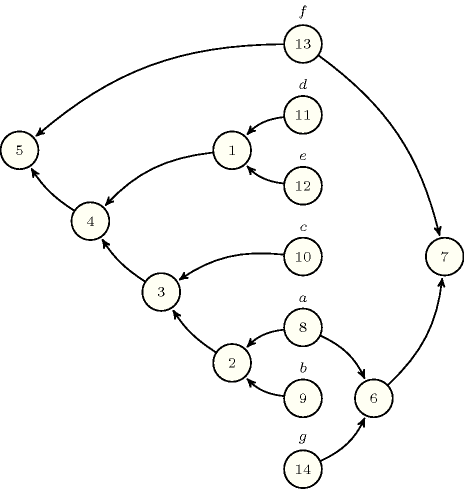##### Figure 5.373.9. Incomparability graph associated with the two trees to merge described by Figure 5.373.1; the two cliques respectively correspond to the leaves of the two trees to merge.